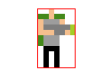# How do I detect a given sprite's bounds automatically using Java?

Background

I have the following png file that contains the player sprite.

As with any png file, the non-colored pixels are transparent.(Width: 16px; Height: 16px)

Currently, I can erect a rectangle (collision-bounds), using java.awt.Rectangle around the image using the image's width and height, as seen below.I would like to crop the image so that the width and height are changed to the edges of the colored portion of the image, much like what is shown below.This will allow for more accurate collision-detection between entities or objects in a game.

I want to construct a method that handles this modification dynamically, as seen below.

//Method calculates how far in from [top, bottom, left and right]
//the [non-zero] or [transparent] pixel-data of an image are
//in order to construct a new image that starts at the edges
//of the colored portion of the image.
{
BufferedImage result = null;

return result;
}


Problem

However, I am uncertain as to the algorithm to use to achieve the result above.

Can anyone give a step-by-step on how to detect these bounds and how to implement such a fuction as described above?

• Do you want to calculate the rectangle, or do you want to crop the image? – Bálint Dec 14 '17 at 23:22
• @Bálint oh yes, sorry, should have specified. I want to crop the image. – Nicholas Adamou Dec 14 '17 at 23:23

You first need to scan the image for the edges. You need to loop through each pixel in the image and store the position of the pixel closest to the left, the pixel closest to the right, etc.

Pixels are stored in the

 ARGB
8 bit alpha | 8 bit red | 8 bit green | 8 bit blue


format in integers. This means that you need to use the

int alpha = (color >> 24) & 0xFF;


method to get the alpha value of a pixel.

int minX = Integer.MAX_VALUE;
int maxX = integer.MIN_VALUE;
int minY = Integer.MAX_VALUE;
int maxY = integer.MIN_VALUE;
for (int x = 0; x < img.getWidth(); x++) {
for (int y = 0; y < img.getHeight(); y++) {
int color = img.getRGB(x, y);
int alpha = (color >> 24) & 0xFF;
if (alpha != 0) {
// This sets the edges to the current coordinate
// if this pixel lies outside the current boundaries
minX = Math.min(x, minX);
maxX = Math.max(x, maxX);
minY = Math.min(y, minY);
maxY = Math.max(y, maxY);
}
}
}


Then you need to create an image and copy the pixel data to it:

BufferedImage result = new BufferedImage(maxX - minX, maxY - minY, BufferedImage.TYPE_INT_ARGB);

for (int x = 0; x < maxX - minX; x++) {
for (int y = 0; y < maxY - minY; y++) {
result.setRGB(x, y, img.getRGB(x + minX, y + minY));
}
}

• Thanks, Bálint, for your answer. Quite through! However, after implementing your solution and testing it. It appears to be going from i.stack.imgur.com/NRRg4.png to i.gyazo.com/44de8f79fc60c96add385140d3e0e204.png. – Nicholas Adamou Dec 14 '17 at 23:58
• This error is occurring because minY and maxY are equal, however, the targeted image within the 16x16px image is not equal in width and height. – Nicholas Adamou Dec 15 '17 at 0:22
• @Nicholas I forgot to change 2 xs to ys. Should be fixed now – Bálint Dec 15 '17 at 9:31